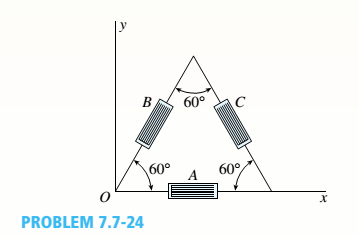# A 600 strain rosette, or delta rosette, consists of three electrical-resistance strain gages arranged as shown in the figure. Gage A measures the normal strain e, in the direction of the x axis. Gages Band C measure the strains and e in the inclined directions shown. Obtain the equations for the strains 8. e. and y, associated with the x-v axes.### Mechanics of Materials (MindTap Co...

9th Edition
Barry J. Goodno + 1 other
Publisher: Cengage Learning
ISBN: 9781337093347
Chapter 7, Problem 7.7.24P
Textbook Problem
292 views

## A 600 strain rosette, or delta rosette, consists of three electrical-resistance strain gages arranged as shown in the figure. Gage A measures the normal strain e, in the direction of the x axis. Gages Band C measure the strains and e in the inclined directions shown. Obtain the equations for the strains 8. e. and y, associated with the x-v axes.To determine

The equation for the strain in the x direction.

The equations for the strain in the y direction.

The equation of the shear strain associated with x y axes.

### Explanation of Solution

The following figure shows the 60 ° strain rosette having three electrical resistance.

b

Figure-(1)

From figure (1)

At resistor A the angle θ is zero and Strain is ε A . At resistor B the angle is 60 ° and strain is ε B . At resistor C the angle is 120 ° and the strain is ε C .

For θ = 0 °

ε x = ε A

Write the expression for the normal strain at an angle of 60 ° from the horizontal.

ε B = ε x + ε y 2 + ε x ε y 2 cos 2 θ + γ x y 2 sin 2 θ .....(I)

Here, the strain at an angle of 60 ° from the horizontal is ε B , the strain in the x direction is ε x , the strain in the y direction is ε y , the shear strain is γ x y and the angle between resistor A and B is θ .

Substitute 60 ° for θ and ε A for ε x in Equation (I).

ε B = ε A + ε y 2 + ε A ε y 2 cos ( 2 × 60 ° ) + γ x y 2 sin ( 2 × 60 ° ) ε B = ε A + ε y 2 ( ε A ε y 4 ) + γ x y 3 4 ε B = ε A 4 + 3 ε y 4 + γ x y 3 4 .....(II)

Write the expression for the normal strain at an angle of 120 ° from the horizontal.

ε C = ε x + ε y 2 + ε x ε y 2 cos 2 θ + γ x y 2 sin 2 θ .....(III)

Here, the strain in the resistor C is ε C .

Substitute 120 ° for θ and ε A for ε x in Equation (III)

### Still sussing out bartleby?

Check out a sample textbook solution.

See a sample solution

#### The Solution to Your Study Problems

Bartleby provides explanations to thousands of textbook problems written by our experts, many with advanced degrees!

Get Started

Find more solutions based on key concepts
What are the three major axes used during CNC mill programming?

Precision Machining Technology (MindTap Course List)

List the advantages of having one ignition coil per cylinder.

Automotive Technology: A Systems Approach (MindTap Course List)

Explain why database design is important.

Database Systems: Design, Implementation, & Management

Take a 500-sheet ream of computer paper as it comes wrapped. Unwrap it and measure the height, width, and lengt...

Engineering Fundamentals: An Introduction to Engineering (MindTap Course List)

Explain what occurs when vendor proposals are tested and evaluated. A(n) _____ test measures the performance of...

Enhanced Discovering Computers 2017 (Shelly Cashman Series) (MindTap Course List)

Why must the weld crater be cleaned before starting a new electrode?

Welding: Principles and Applications (MindTap Course List)

If your motherboard supports ECC DDR3 memory, can you substitute non-ECC DDR3 memory?

A+ Guide to Hardware (Standalone Book) (MindTap Course List)